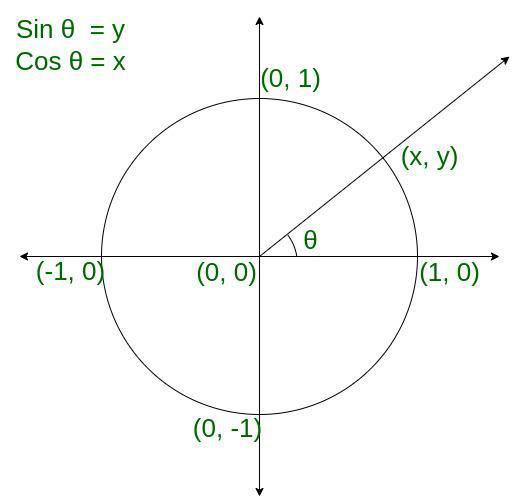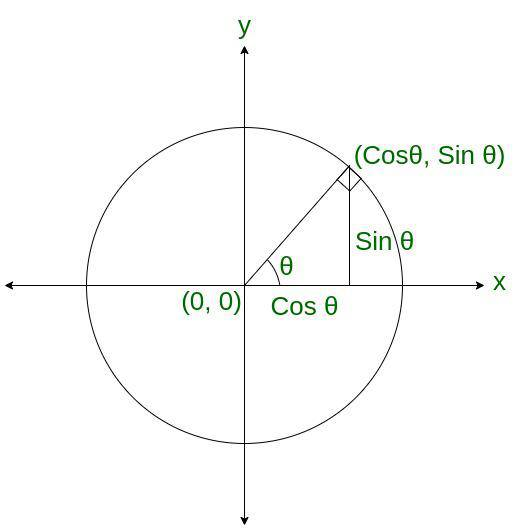GFG App
Open AppBrowser
Continue

# How to use the Unit Circle in Trigonometry?

A unit circle is defined as a circle whose radius is 1 unit, the centre is at the origin, and the circumference is 2π, since r = 1. Here, the arc length is equivalent to the measure of the central angle intercepting that arc. The trigonometric functions, sine and cosine have special importance in consideration for the unit circle. For instance, for any point on the terminal of the circle of an angle in standard position, the sine property value of such an angle is equivalent to the y-coordinate of the point. Also, the cosine of such an angle is equivalent to the x-coordinate of that point respectively.Unit Circle in trigonometry

## Unit Circle Formula

The following is the generic equation for a circle:

(x – h)2 + (y – k)2 = r2

where,

• r is the radius of the circle
• (h, k) is the centre coordinates

## Trigonometric Functions Using a Unit Circle

The application of the Pythagoras theorem in a unit circle can be better used to understand trigonometric functions. For this, we consider a right triangle to be placed inside a unit circle in the cartesian coordinate plane. If we notice, the radius of this circle denotes the hypotenuse of the right-angled triangle. The radius of the circle forms a vector. This leads to the formation of an angle, say θ with the positive x-axis. Let us suppose x to be the base length and y to be the altitude length of the right triangle respectively. Also, the coordinates of the radius vector endpoints are (x, y) respectively.

The right-angle triangle holds the sides 1, x, and y respectively. The trigonometric ratio can be computed now, as follows:Now,

sin θ = y,

cos θ = x

Computing, tan θ = sin θ /cos θ = y/x.

On substituting the values of θ, we can obtain principal values as well. All other trigonometric functions’ values can be computed similarly.Trigonometric representation of the unit circle

## Unit Circle with Sin, Cos and Tan ratios

Any point on the unit circle with the coordinates (x, y), which are equal to the trigonometric identities of (cosθ, sinθ). The coordinates of the radius corners represent the cosine and the sine of the θ values for a particular value of θ and radius line. We have cos θ = x, and sin θ = y. There are four parts of a circle each lying in one quadrant, making an angle of 90º,180º, 270º, and 360º. The radius values lie between -1 to 1 respectively. Also, the sinθ and cosθ values lie between 1 and -1 respectively.

### Unit Circle and Trigonometric Identities

The unit circle trigonometric identities for cotangent, secant, and cosecant can be computed using the identities for sin, cos, and tan. Conclusively, we obtain a right-angled triangle with the sides 1, x, and y respectively. Computing the unit circle identities can be expressed as,

sin θ =cos θ =tan θ =sec θ =cosec θ =cot θ =### Unit Circle Table

The trigonometric ratios used in the unit circle table are used to list the coordinates of the points on the unit circle that correspond to common angles.

We can find the secant, cosecant, and cotangent functions also using these formulas:

secθ =cosecθ =cotθ =We have discussed the unit circle for the first quadrant. Similarly, we can extend and find the radians for all the unit circle quadrants. The numbers 1/2, 1/√2, √3/2, 0, and 1 repeat along with the sign in all 4 quadrants.

## Solved Examples on Unit Circle Formula

Problem 1: Prove that point Q is on the unit circle.Solution:

According to the unit circle equation, which is:

x2 + y2 = 1Hence,.

Therefore, we conclude the point Q does not fall on the unit circle.

Problem 2: Compute tan 30o using the sin and cos values of the unit circle.

Solution:

Computing tan 30o using sin and cos values,

Since,Therefore,

We have,

sin 30ocos 30oTherefore,Conclusively,

Tan 30oProblem 3: Validate if the point P(2/3, 3/2) lies on the unit circle.

Solution:

According to the unit circle equation, which is:

x2 + y2 = 1

Here we have

x = 2/3

y = 3/2

Now,

Substituting the values of x and y in the equation

= (2/3)2 + (3/2)2 = 1

= 4/9 + 9/4 = 1

= ((4*4)+(9*4))/36 = 1

= (16 +36)/36 = 1

= 52/36 = 1

= 13/9 = 1

Thus, 13/9 ≠ 1

Therefore, point P does not lie on the unit circle.

Problem 4: Check if the point Q(1/3, 1) lies on the unit circle.

Solution:

According to the unit circle equation, which is:

x2 + y2 = 1

Here we have

x = 1/3

y = 1

Now,

Substituting the values of x and y in the equation

=> (1/3)2 + (1)2 = 1

=> 1/9 + 1 = 1

=> 10/9 = 1

Thus, 10/9 ≠ 1.

Therefore, point Q does not lie on the unit circle.

## FAQs on Unit Circle Formula

Question 1. What is meant by the term unit circle?

Solution:

A unit circle is defined as the location of a point one unit away from a fixed point. It has a center at (0,0) and value of its radius is 1.

Question 2. What is the significance of a unit circle in trigonometry?

Solution:

The unit circle is useful because it lets us solve for the sine, cosine, or tangent of any number of degrees or radians. Knowing the pie chart is especially useful when a specific trigonometry value ​has to be found.

Question 3. How can we check if a point lies on the unit circle or not?

Solution:

Any point lying in a 2D plane that is of the form (x, y) is put in the unit circle equation x2 + y2 = 1 to verify if it lies on the circle or not.

Question 4. What is the value of sin θ cos θ for a point (x, y) on a unit circle?

Solution:

As for a point (x, y), sin θ = y and cos θ = x, the value of sin θ cos θ becomes xy.

My Personal Notes arrow_drop_up